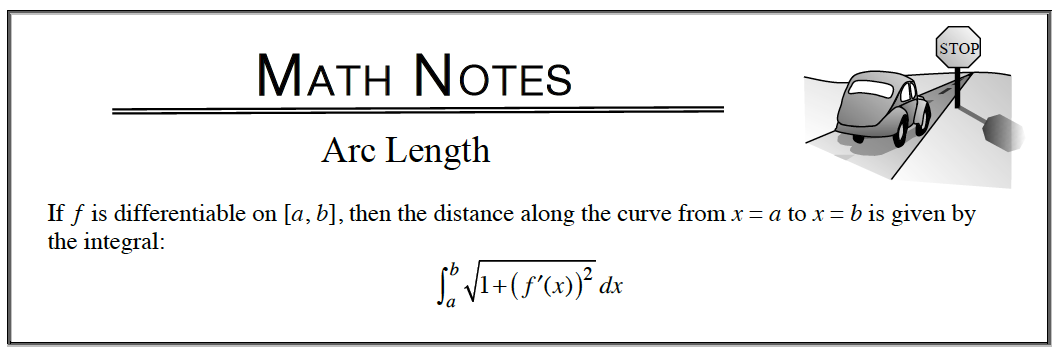### Home > APCALC > Chapter 8 > Lesson 8.4.1 > Problem8-139

8-139.

Express the arc length of each of the following curves as an integral, then evaluate: Homework Help ✎

1. $f (x) = e^x$, from $x = -2$ to $x = 2$

2. $f (x) = \ln(x)$, from $x = 1$ to $x = e$